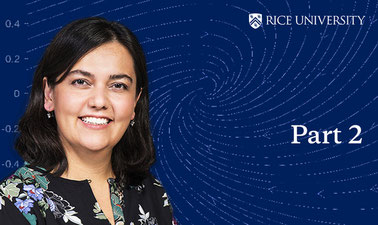# Ordinary Differential Equations and Linear Algebra - Part 2

Study of ordinary differential equations (e.g., solutions to separable and linear first-order equations and to higher-order linear equations with constant coefficients, systems of linear differential equations, the properties of solutions to differential equations) and linear algebra (e.g., vector spaces and solutions to algebraic linear equations, dimension, eigenvalues, and eigenvectors of a matrix), as well as the application of linear algebra to first-order systems of differential equations and the qualitative theory of nonlinear systems and phase portraits.### There is one session available:

After a course session ends, it will be archived.
Starts Jul 26
Ends Dec 31
Estimated 7 weeks
2–8 hours per week
Self-paced
Free

If you are curious about how we model a phenomena from nature and how we understand its behavior from past to future mathematically, this is the course that will give you answers.

This course provides a comprehensive qualitative and quantitative analysis of ordinary differential equations and linear algebra. This course is divided in two parts to be able to facilitate the learning experience. The first part focuses on 1st order differential equations and linear algebra. The second part is about higher order equations that presents beautiful theory of linearity, as well as the complexity of nonlinearity.

### At a glance

• Institution: RICEx
• Subject: Math
• Level: Intermediate
• Prerequisites:

This course require single variable calculus, differentiation and integration.

• Language: English
• Video Transcript: English

# What you'll learn

Skip What you'll learn
• Higher order linear differential equations with constant coefficients:
• Nonhomogeneous Analysis
• Eigenvalues-Eigenvectors
• Systems of Differential Analysis
• Comprehensive Analysis on Stability behavior of 2x2 linear systems
• Nonlinear System analysis by Phase Plane portraits
• Nonlinear System analysis by Linearization